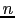# Homework IV

Tom Kelliher, CS 240

25 points, due Mar. 7

1. (10 pts.) 3-40. Use only the logic building blocks mentioned in the problem.

2. (10 pts.) 4-11. Hint: This is a circuit with eight inputs. You probably dont't want to construct the truth table for this circuit. Instead, consider a modular divide-and-conquer approach, similar to how one uses divide-and-conquer when designing an-bit adder fromone-bit full adders. Your one-bit module will have three single-bit inputs and one single-bit output; you'll have to decide what the inputs and output represent. You will need to carefully consider whether the module outputs propagate from the least significant bit toward the most significant bit (a la addition) or in the opposite direction.

3. (10 pts.) Design two 64-bit adders, including figures showing the connections between components:
How would the delays for your 64-bit adders generalize to an-bit adders?